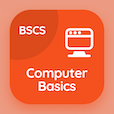Colleges Online Courses

College Math Quizzes

College Math Quiz PDF - Complete

# Composition of functions Quiz Questions Online p. 4

Learn Composition of functions quiz questions and answers, composition of functions MCQ with answers PDF 4 to study College Math course online. Functions and Limits trivia questions, composition of functions Multiple Choice Questions (MCQ) for online college degrees. "Composition of functions Quiz" PDF Book: complex numbers, trigonometric ratios of allied angles, composition of functions test prep for best online ACT prep class.

"If ƒ(x) = xsecx, then ƒ(0) =" MCQ PDF: 0, −1, 1, and √(2) for GRE subject tests. Study functions and limits questions and answers to improve problem solving skills for online bachelor degree programs.

## Quiz on Composition of functions MCQs

MCQ: If ƒ(x) = xsecx, then ƒ(0) =

−1
0
1
√(2)

MCQ: If tanθ < 0, sinθ < 0, then the terminal arm of the angle lies in the quadrant

1
2
3
4

MCQ: If z1 = 2 + ι, z2 = 1 + 3ι, then ι Re ( z1 - z2 ) =

1
ι
2 ι
2

MCQ: Cos(π+α) =

cosα
−cosα
−sinα
sinα

MCQ: ƒ(x) = sin(x) is

linear function
odd function
even function

### More Quizzes from College Math Course